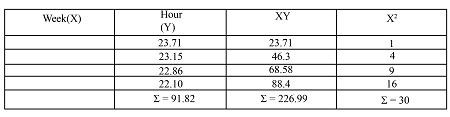# We have been given the machine hour history below. You have been asked to calculate a linear...

## Question:

We have been given the machine hour history below. You have been asked to calculate a linear trend line for this data.

What is the intermediate solution for the value of {eq}\sum {/eq}(ty)ty?

WeekHours
123.71
223.15
322.86
422.10

## Regression

It is a forecasting tool use in long-term forecasting by using measurable data for an equation. Regression is adopted both in business and environmental studies. It helps in finding out the relationship between the dependent and independent variables as well.

## Answer and Explanation:

Become a Study.com member to unlock this answer! Create your account

Given,

Assigning Week as independent variable X and Hours as dependent on Week(X):a) Trend line Formula:

{eq}Y = a + bX {/eq}

Where,

{eq}\...

See full answer below.

#### Learn more about this topic:Simple Linear Regression: Definition, Formula & Examples

from

Chapter 8 / Lesson 2
32K

Simple linear regression is a great way to make observations and interpret data. In this lesson, you will learn to find the regression line of a set of data using a ruler and a graphing calculator.